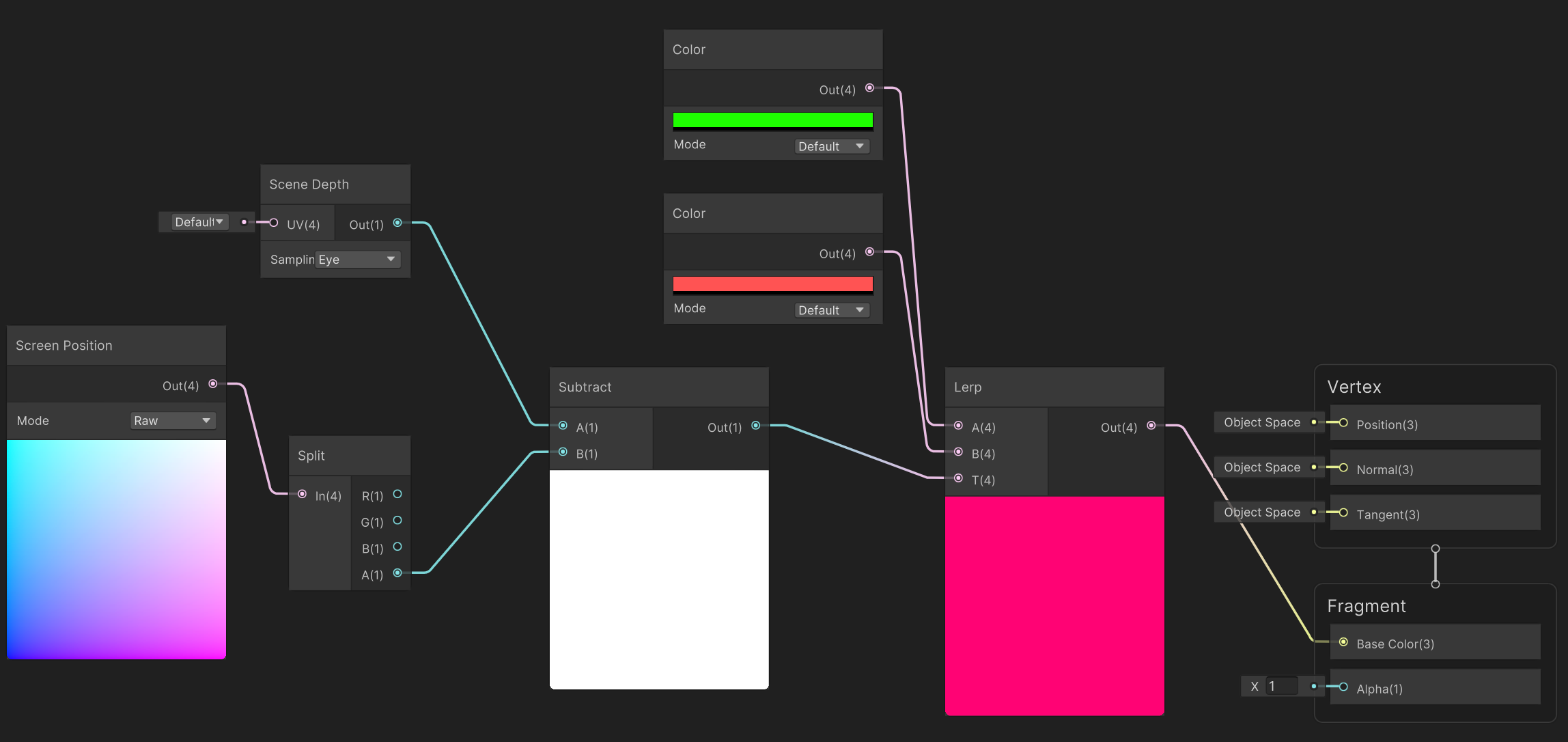# Understanding Depth Intersections with a Shader

To colour the intersection between two objects in a shader without passing into the shader the geometry of a bunch of models takes a bit of trickery because once an object has been rendered information about its geometry is lost.  This is because the object becomes pixels on the screen.

Shaders basically work with floating float3 or float4 values which in the end must represent a colour.

Shaders also only process one pixel at a time.  That means when executing the shader, it only has pixel information available to it based on a single fragment.  And it's really only this fragment that the shader can access any position data for.

In my Shader Graph course, we create a simple shader to produce a rimmed effect where two objects overlap each other (as shown in the picture of the sphere embedded in the plane above).

The shader is applied to the sphere only and therefore colours the sphere only.  But it colours the sphere with black and white values based on the depth of other objects behind it.  But how?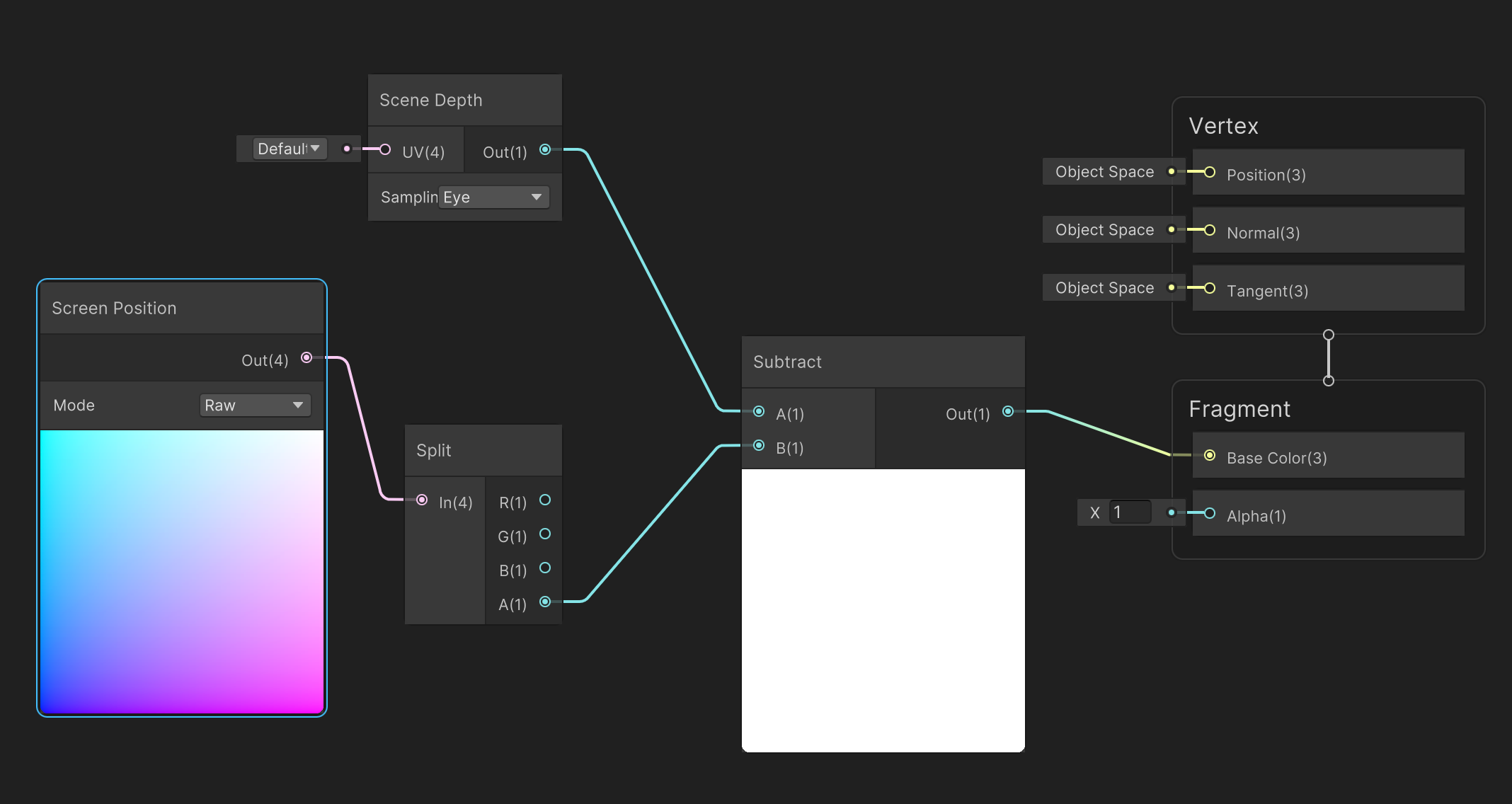Above is the shader used to calculate the differences in depth and set the pixels colours of the sphere in values ranging from black (where the sphere is closest to the plane) and white (where it is furthest).  The two nodes used are Scene Depth and Screen Position.  This shader is set to transparent so the object gets drawn after any opaque objects.

The pixel colour is set to be the scene depth value minus the alpha (or w) value of the screen position.  If you consider the values coming out of each node then you can make the following conclusions as shown in this next image.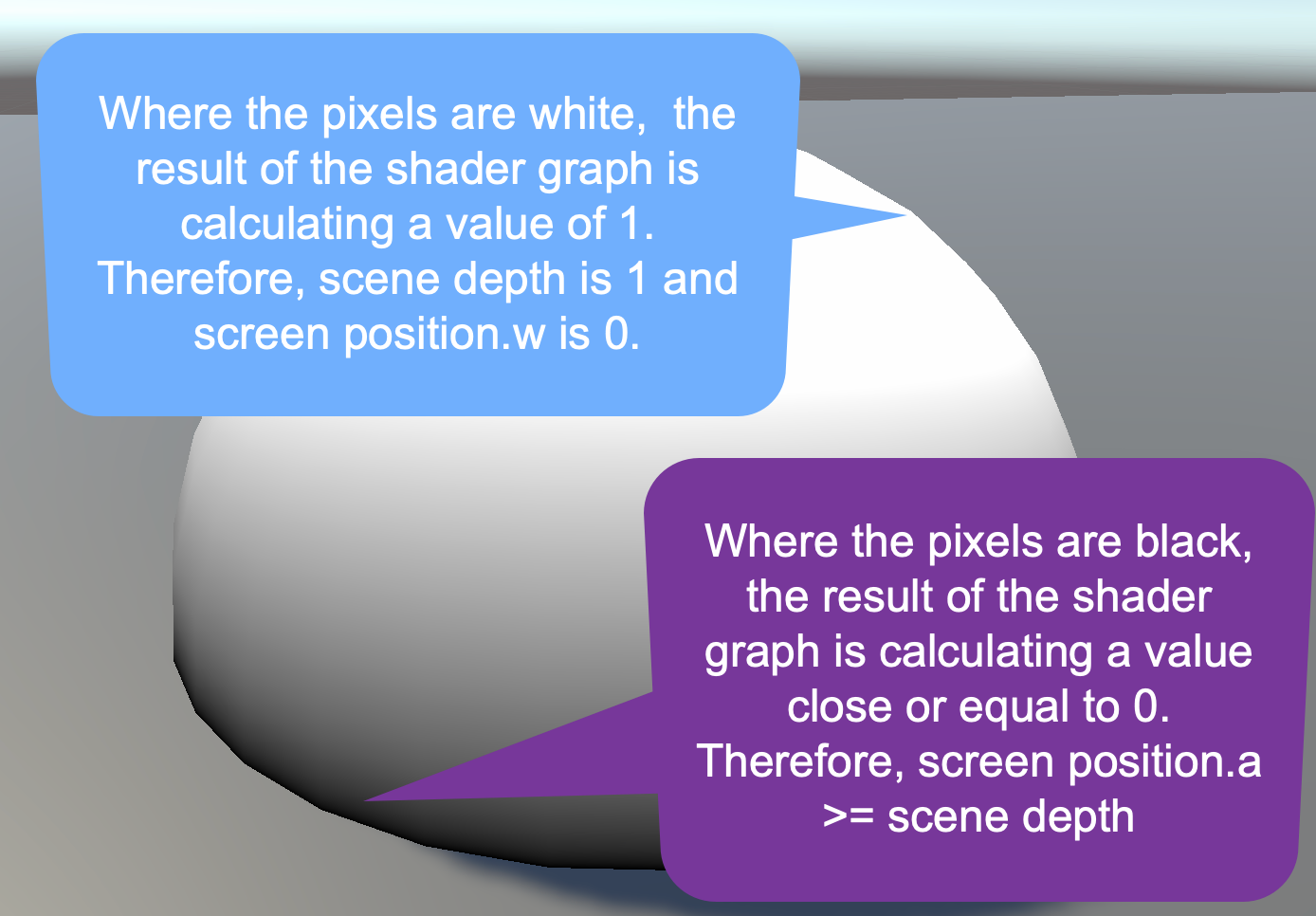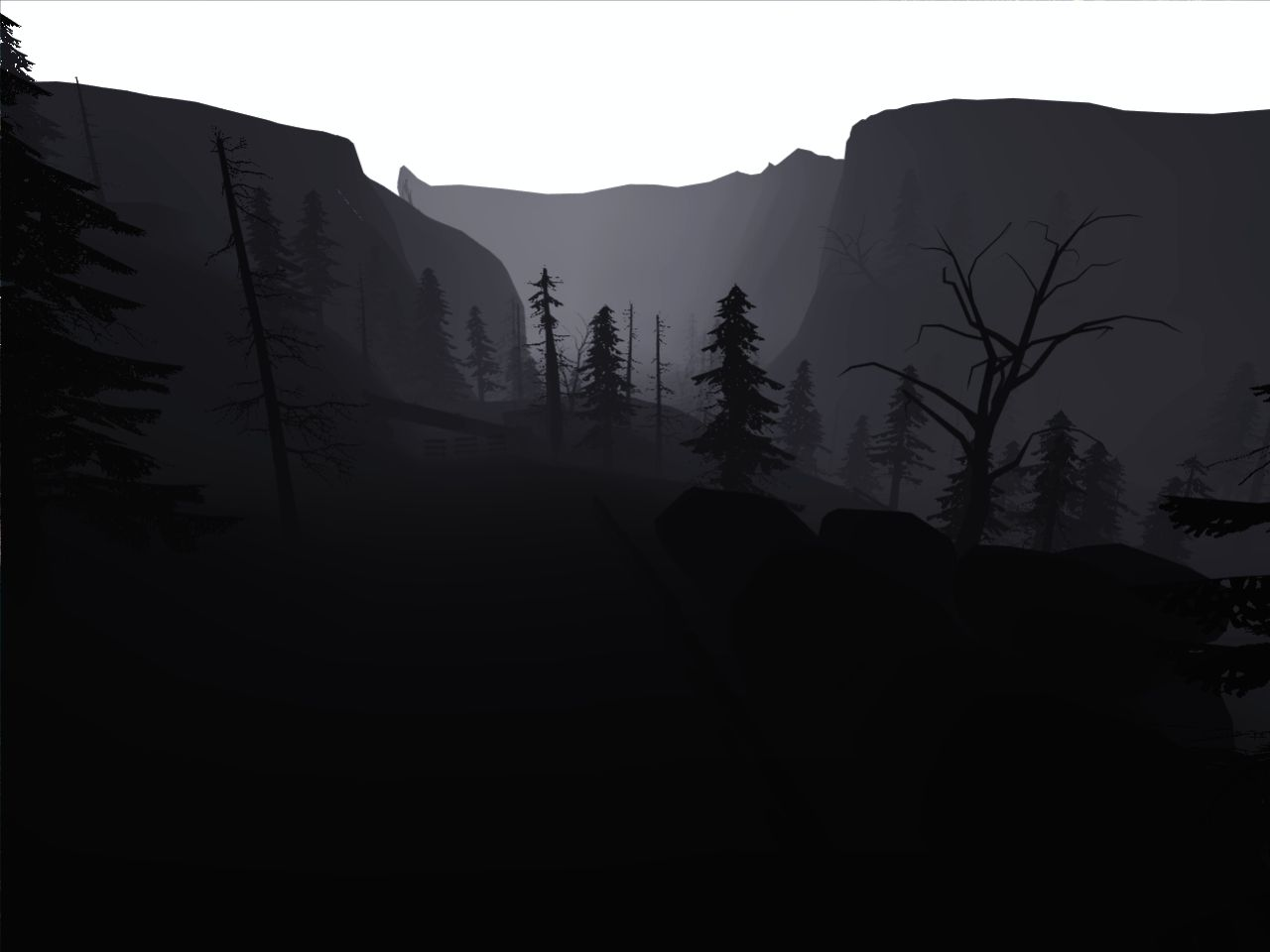So how does this shader work?  The Scene Depth node gets the depth of the scene at the currently being processed pixel location.  This depth value is taken from the z-buffer or depth buffer.  As every object in the scene is drawn, it's normalised depth in the scene is recorded in a grey scale image.  Furtherest points from the camera are recorded as 1 (white) and closest points 0 (black).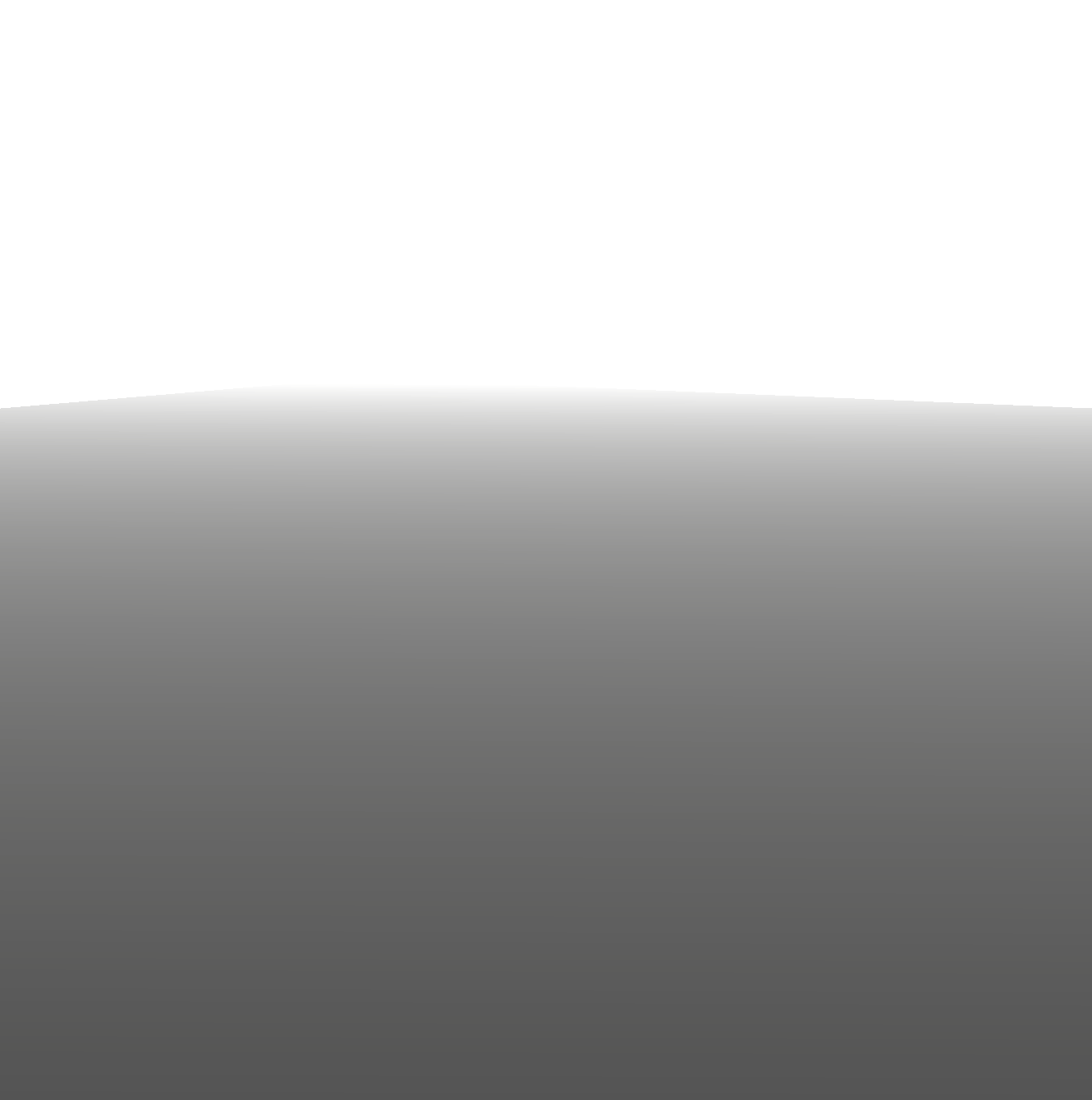In the simple scene of the plane and sphere, the plane is the first thing drawn as it is an opaque object. This results in a depth buffer that looks like this.

When the sphere is drawn, the screen position depth, which is the w (same as alpha) value of the float4.
Because the sphere is not yet drawn in the depth buffer, it's depth value can't be taken from there.  Instead, the raw screen depth is used as the w value is essentially the depth of the sphere pixel (normalised).

By using a subtract node in the shader to take the screen position depth away from the z buffer depth, we are getting the difference in the depth at any pixel location.  The larger the gap in depth, the closer to 1 the final value will calculate to and produce a colour close to white.  The smaller the gap, the closer to 0 and the colour will be black.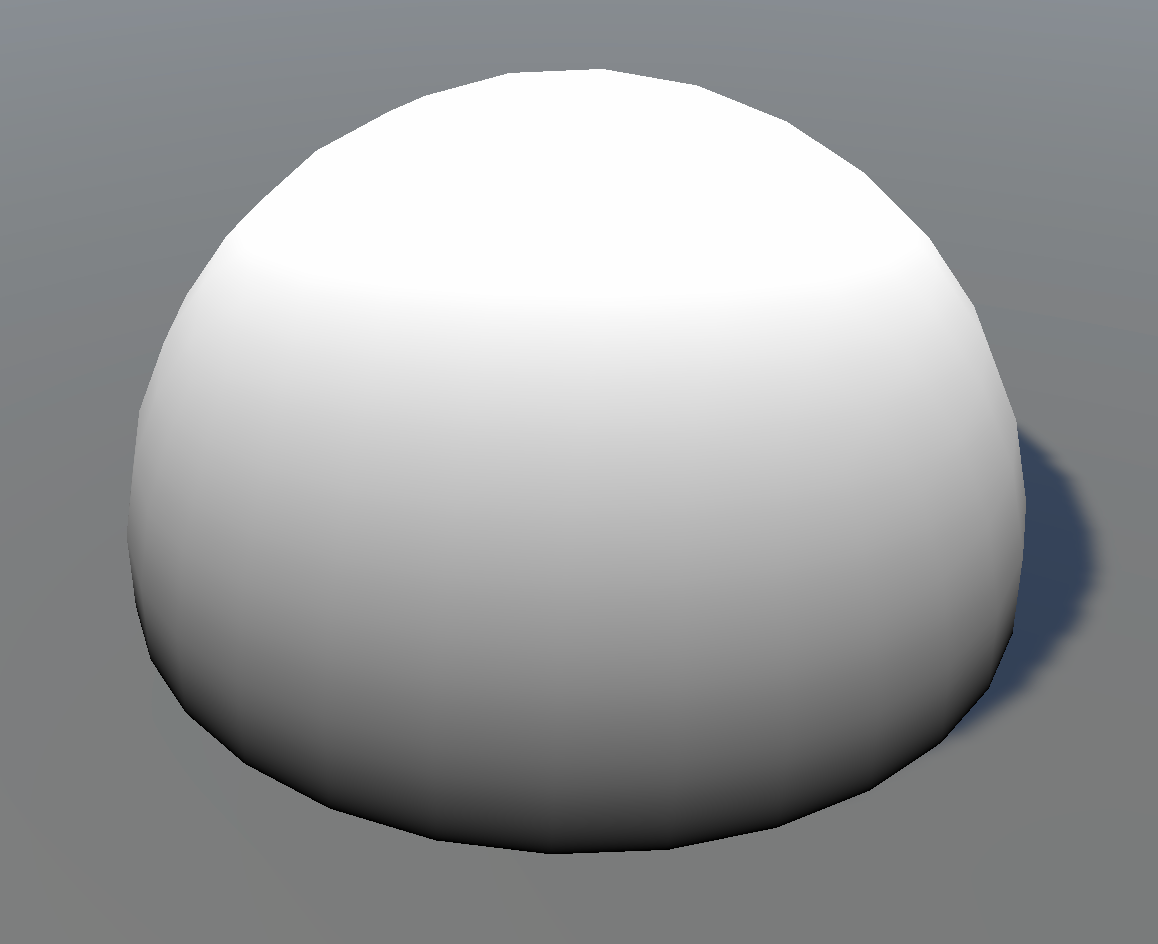In that way we can produce this rimmed effect where two objects are close to each other with respect to their depth from the camera.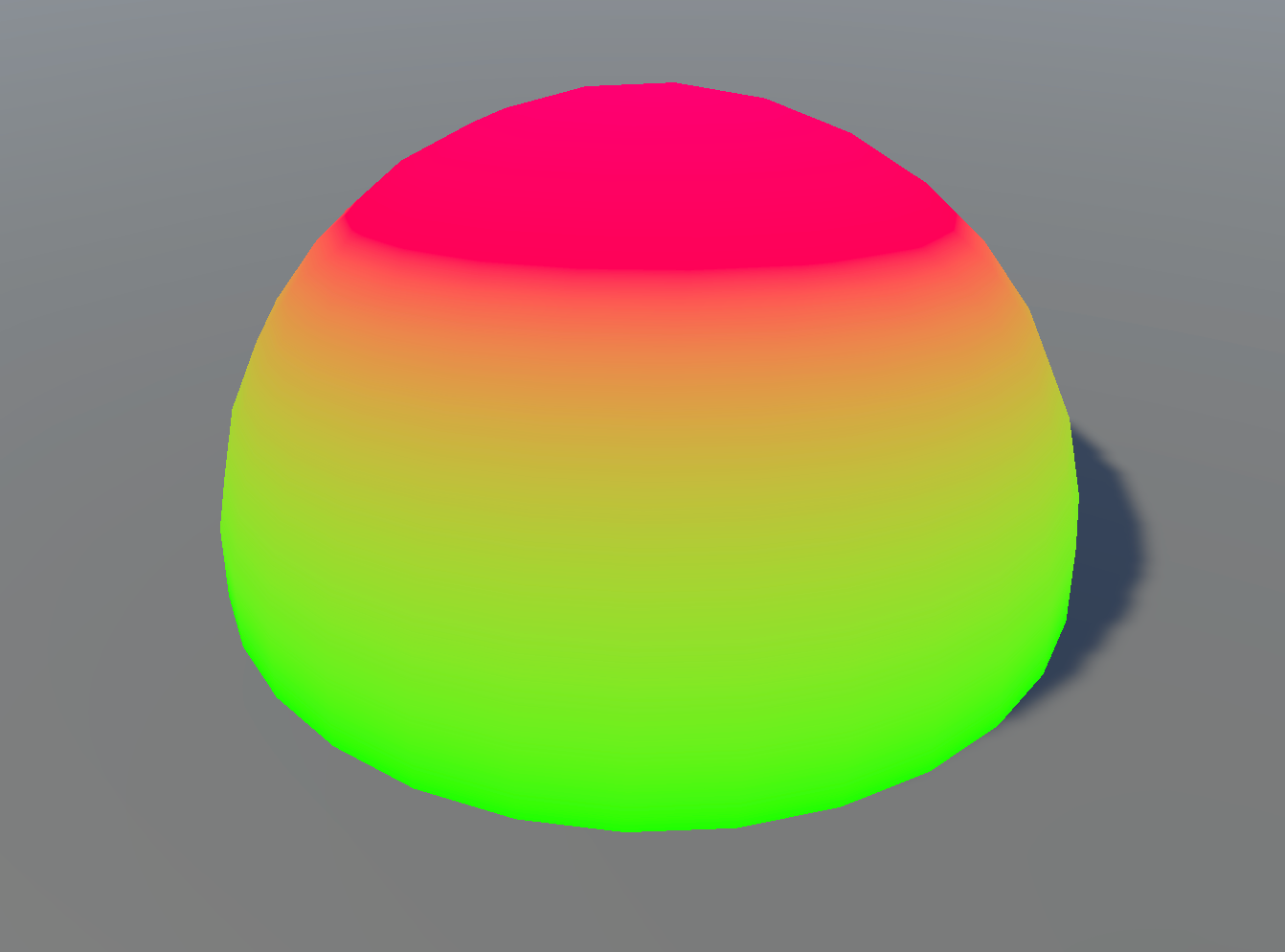This this black and white depth colouring result, you can then use it in a lerp node to change the colours.

The shader graph can be modified to include colours like this: